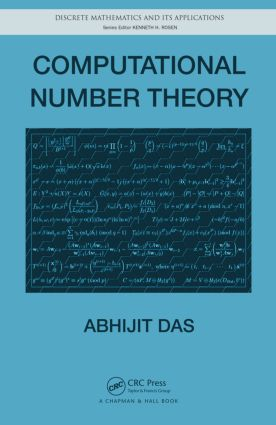Computational Number Theory

1st Edition

Chapman and Hall/CRC

614 pages | 13 B/W Illus.

Purchasing Options:\$ = USD
Hardback: 9781439866153
pub: 2013-03-18
SAVE ~\$23.00
Currently out of stock
\$115.00
\$92.00
x
eBook (VitalSource) : 9780429090516
pub: 2016-04-19
from \$57.50

FREE Standard Shipping!

Description

Developed from the author’s popular graduate-level course, Computational Number Theory presents a complete treatment of number-theoretic algorithms. Avoiding advanced algebra, this self-contained text is designed for advanced undergraduate and beginning graduate students in engineering. It is also suitable for researchers new to the field and practitioners of cryptography in industry.

Requiring no prior experience with number theory or sophisticated algebraic tools, the book covers many computational aspects of number theory and highlights important and interesting engineering applications. It first builds the foundation of computational number theory by covering the arithmetic of integers and polynomials at a very basic level. It then discusses elliptic curves, primality testing, algorithms for integer factorization, computing discrete logarithms, and methods for sparse linear systems. The text also shows how number-theoretic tools are used in cryptography and cryptanalysis. A dedicated chapter on the application of number theory in public-key cryptography incorporates recent developments in pairing-based cryptography.

With an emphasis on implementation issues, the book uses the freely available number-theory calculator GP/PARI to demonstrate complex arithmetic computations. The text includes numerous examples and exercises throughout and omits lengthy proofs, making the material accessible to students and practitioners.

Reviews

"This book would be a good choice for cryptography and engineering students wanting to learn the basics of algorithmic number theory."

Mathematical Reviews, November 2014

Arithmetic of Integers

Basic Arithmetic Operations

GCD

Congruences and Modular Arithmetic

Linear Congruences

Polynomial Congruences

Multiplicative Orders

Continued Fractions

Prime Number Theorem and Riemann Hypothesis

Running Times of Arithmetic Algorithms

Arithmetic of Finite Fields

Existence and Uniqueness of Finite Fields

Representation of Finite Fields

Implementation of Finite Field Arithmetic

Some Properties of Finite Fields

Alternative Representations of Finite Fields

Computing Isomorphisms among Representations

Arithmetic of Polynomials

Polynomials over Finite Fields

Finding Roots of Polynomials over Finite Fields

Factoring Polynomials over Finite Fields

Properties of Polynomials with Integer Coefficients

Factoring Polynomials with Integer Coefficients

Arithmetic of Elliptic Curves

What Is an Elliptic Curve?

Elliptic-Curve Group

Elliptic Curves over Finite Fields

Some Theory of Algebraic Curves

Pairing on Elliptic Curves

Elliptic-Curve Point Counting

Primality Testing

Introduction to Primality Testing

Probabilistic Primality Testing

Deterministic Primality Testing

Primality Tests for Numbers of Special Forms

Integer Factorization

Trial Division

Pollard’s Rho Method

Pollard’s p - 1 Method

Dixon’s Method

CFRAC Method

Cubic Sieve Method

Elliptic Curve Method

Number-Field Sieve Method

Discrete Logarithms

Square-Root Methods

Algorithms for Prime Fields

Algorithms for Fields of Characteristic Two

Algorithms for General Extension Fields

Algorithms for Elliptic Curves (ECDLP)

Large Sparse Linear Systems

Structured Gaussian Elimination

Lanczos Method

Wiedemann Method

Block Methods

Public-Key Cryptography

Public-Key Encryption

Key Agreement

Digital Signatures

Entity Authentication

Pairing-Based Cryptography

Appendix A: Background

Appendix B: Solutions to Selected Exercises

Index

Abhijit Das

Kharagpur, West Bengal, India

Abhijit Das is an associate professor in the Department of Computer Science and Engineering at the Indian Institute of Technology, Kharagpur. His research interests are in the areas of arithmetic and algebraic computations with specific applications to cryptology.